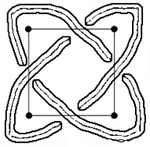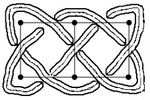Celtic Knotwork : the ultimate tutorial

# How many strings ?

Toutes les versions de cet article : [français] [français]

Instead a of mere strings, we are going to count the number of connected components of our knot, it sounds way more fashionable at a party.

So we’re asking how many connected components there will be in our knot once we’ve gone through the tedious task of sorting it all out. How many pens do I need in order for a single string to remain of the same unique colour throughout its whole trip ?If you take a square, with p vertices on one side, you’ll have p different strings, going up-right diagonally to the North-East, then bouncing at the top row, rushing down to the South-East, then bouncing on the right side and going South-West, bouncing again on the bottom row leading North-West, then on the left column and back to North-East. The square with 2 vertices will have 2 simple interlaced strings.If you take a simple line of squares, that is 2 vertices vertically and n horizontally, how many do you get ? If you have 2 vertices, that is a simple square, we just saw that you get 2. If you had only 2 vertices vertically in a single row, you would get a figure 8, that is a single string. With 3 vertices wide, you get one single string. So that is, 1, 2, 1, 2... whether 1 or 2 depending on the parity of n.

Now, if you have a rectangle with pxq vertices, you’ll get...

Tell me, what can you get out of two integer numbers ? Something meaningful, something which out of (2,n) gives 1 or 2 depending whether n is odd or even, and which out of (p,p) gives p.

That’s... the Greatest Common Divisor of p and q.

The number of strings of a rectangle of pxq vertices is the greatest common divisor of p and q. For example 12x9 will yield 3 strings because 12=4x3 and 9=3x3. Take 4x5, and you’ll get a single string because 4 and 5 don’t have common divisors (except 1), they are relatively prime. That will happen whenever you have prime numbers (and that the second is not a multiple of the first), for example 6353x8527 will have a unique, very very long string ! You can look for prime numbers here.

If you take triangles, it’s almost the same : an equilateral triangle with p vertices on its side will have (p+1)÷2 strings. The triangle, with 2 vertices, is associated with the trefoil knot.

Things get more complicated when you introduce walls : a new wall forbidding a crossing between two disjoint strings will actually fuse them into one ! Whereas a wall breaking a self-crossing can do two things, depending on the type of wall, transversal or across, it splits the string in two or it doesn’t.

Therefore it is easy to tailor the number of strings to your needs : do as you want and at the end, put some inconspicuous walls here and there to split or join the strings as you wish.

An interesting question that I, as a researcher in statistical mechanics, can not fail to ask myself is : given a probability for an edge to become a wall, like in the percolation or dimer models, what is the distribution of the connected components, its average number, the average size of a typical component ?

Répondre à cet article

dimanche 8 novembre 2009, par